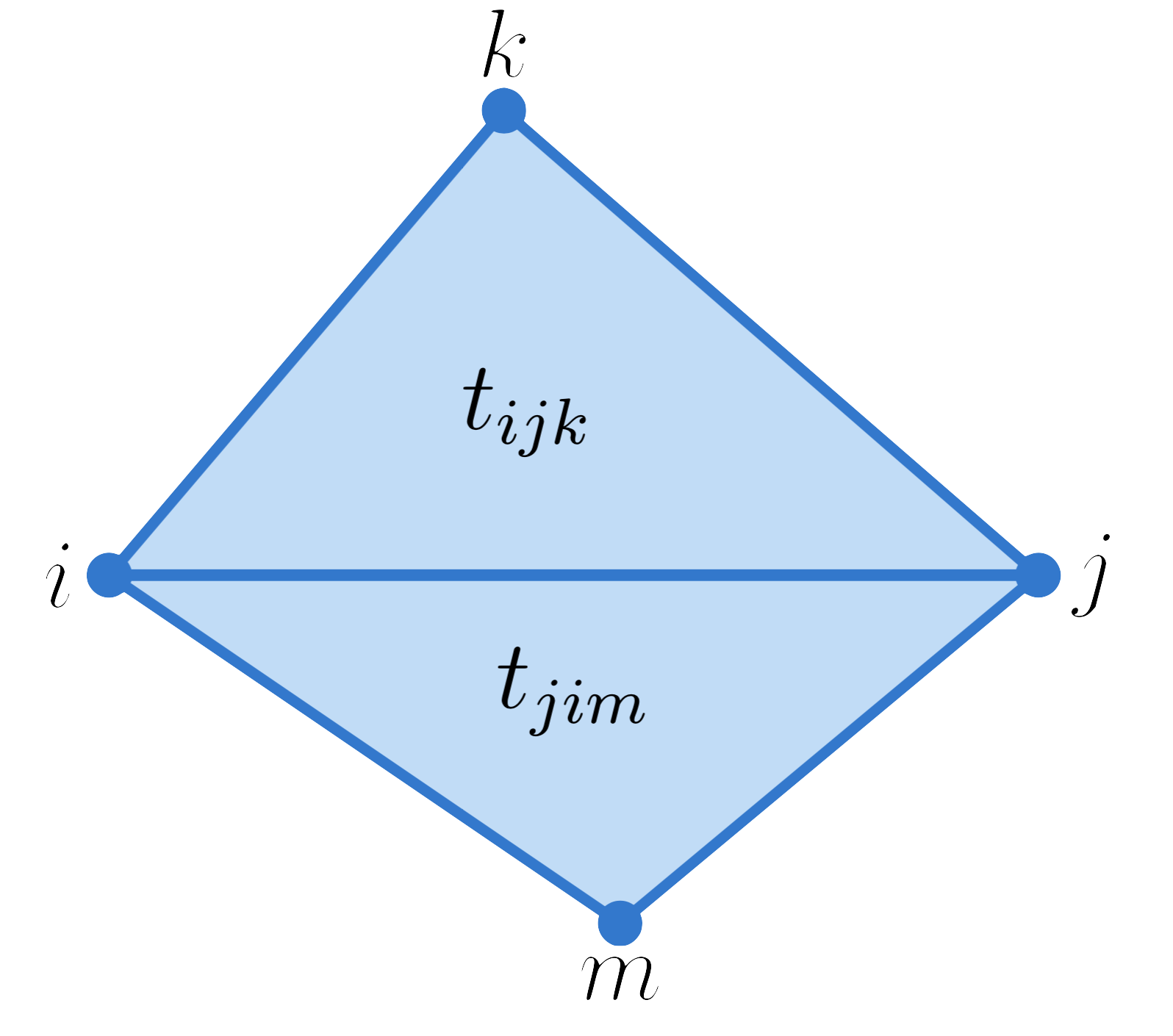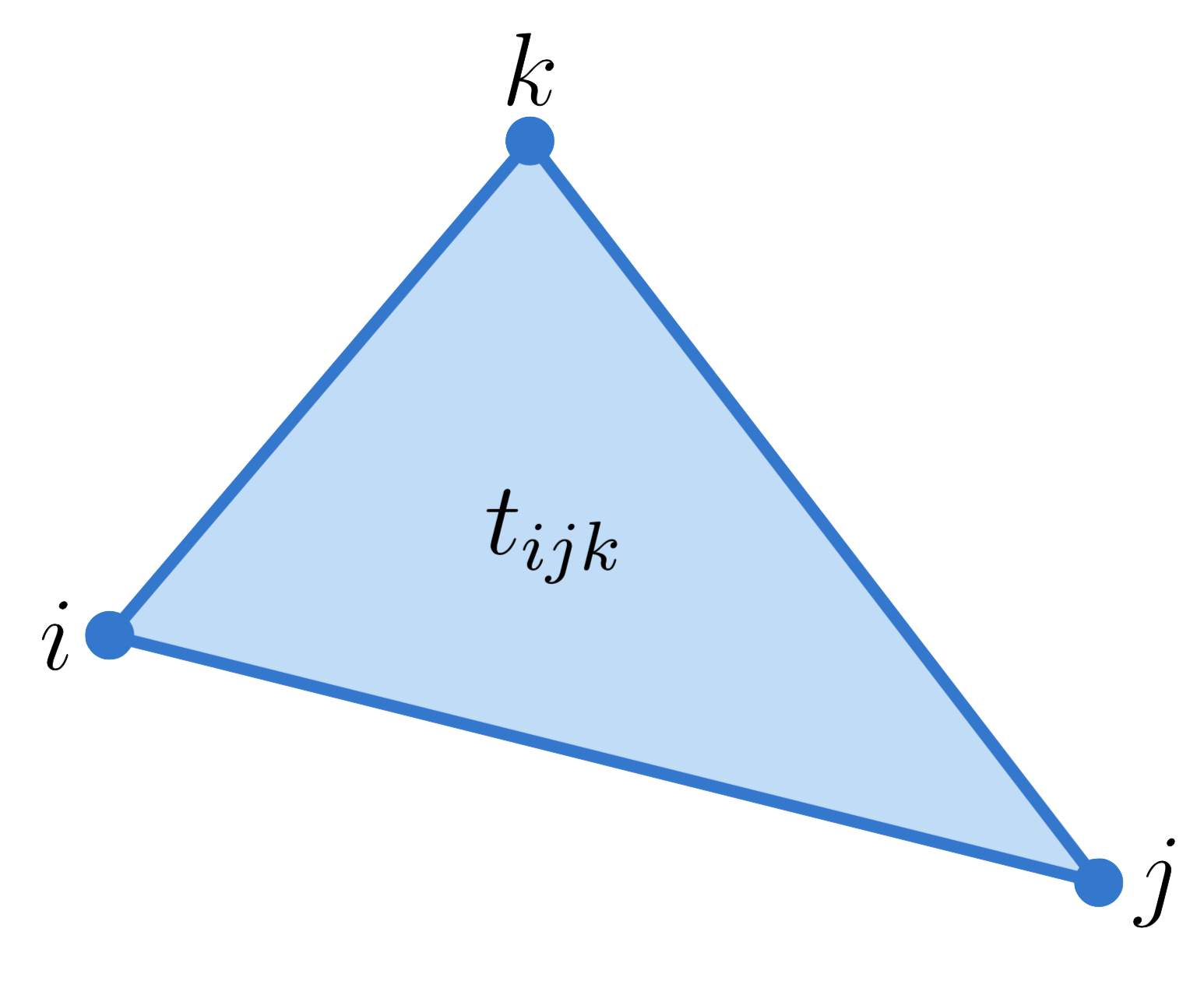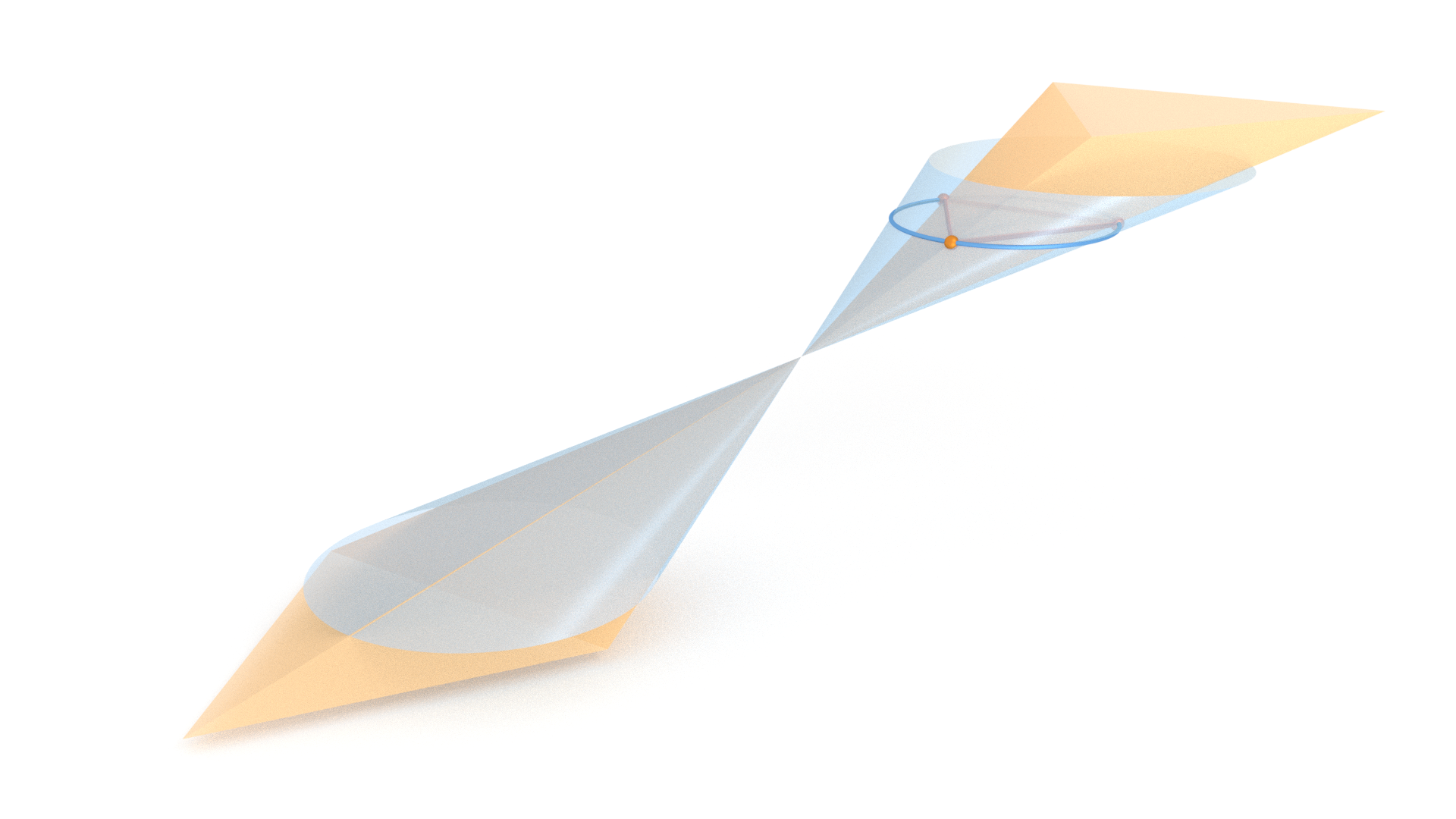Notes following Conformal Equivalence of Triangle Meshes by Springborn, Schroeder and Pinkall.

## Conformal equivalence in the smooth setting

Two metrics $g, \tilde g$ on a manifold $M$ are conformally equivalent if $g = e^{2u} \tilde g$ for some $u:M \to \R$. Earlier, I showed that the conformal equivalences of $\C$ with $\C$ are given by holomorphic or antiholomorphic functions. I think it follows that the conformal equivalences of the Riemann sphere are given by the Möbius transformations.

### Cross Ratios

First, we will define cross ratios for points in $\hat \C$.

Given points $a, b, c, d \in \hat \C$, there exists a unique Möbius transformation $\phi$ such that $\phi(a) = \infty$, $\phi(b) = 1$ and $\phi(c) = 0$. The cross ratio of $a,b,c,d$, denoted $[a,b;c,d]$ is simply $\phi(d)$.
$[a,b;c,d] = \frac{(b-a)(d-c)}{(b-c)(d-a)}$
Proof

Let $\phi(z) = \frac{z-c}{z-a}\frac{b-a}{b-c}$. Note that $\phi(a) = \infty$, $\phi(b) = 1$, and $\phi(c) = 0$. Thus $\phi(d) = [a,b;c,d]$. Evaluating $\phi(d)$, we see that it is the desired ratio.

Taking this equation as the definition of the cross ratio, we can extend the concept of cross ratio to general Riemannian manifolds.

## Discrete Conformal Equivalence

In the discrete setting, a "discrete manifold" $M$ is simply a triangle mesh $M = (V, E, T)$

A discrete metric on $M$ is a function $\ell:E \to \R_{\geq 0}$ satisfying the triangle inequality along the edges of each triangle.
Two discrete metrics $\ell, \tilde\ell$ on $M$ are discretely conformally equivalent if there exists a map $u:V \to \R$ such that $\tilde \ell_{ij} = e^{(u_i + u_j)/2} \ell_{ij}$

In the following, it will be convenient to work with the logarithm of the metric. Let $\lambda_{ij} := 2 \log \ell_{ij}$. Note that this turns the definition of conformal equivalence into a linear equation

$\tilde \lambda_{ij} = \lambda_{ij} + u_i + u_j$

One nice property of this definition of conformal equivalence is that it interacts nicely with Möbius transformations of our ambient space (i.e. applying a Möbius transformation to the ambient space produces a conformally equivalent discrete manifold, as it should).

Let $f$ be a Möbius transformation on $\C^n$. Then there exists a real-valued function $\rho:\C^n \to \R^n$ such that for all pairs $x,y \in \C^n$ we have $\|f(x)-f(y)\| = \rho(x)\;\rho(y)\;\|x-y\|$
Since Möbius transformations wre compositions of translation, rotations, scalings, and inversions of spheres, it suffices to verify the proposition for each of these cases separately. Translations and rotations preserve distances, and scaling changes all distances by a multiplicative constant, so the proposition clearly holds in these cases. Now, we just have to check the proposition when $f$ is an inversion of a sphere. Since we have already verified the proposition for translation and scaling, it suffices to check an inversion of the unit sphere (i.e. $f(z) = \frac 1z$). \begin{aligned} \left\|f(x) - f(y)\right\| &= \left\|\frac 1x - \frac 1y\right\|\\ &= \left\|\frac{y-x}{yx}\right\|\\ &= \frac 1 {\|x\|} \frac 1 {\|y\|} \|x-y\| \end{aligned}

Suppose $M$ is embedded in $\R^{2n} \cong \C^n$, and equip $M$ with the induced discrete metric. By the proposition, we see that $M$ is discretely conformally equivalent to its image under any Möbius transformation of $\C^n$.

The cross ratio of an edge $e_{ij}$ between triangles $t_{ijk}$ and $t_{jim}$ is defined to be $\cr_{ij} := \frac{\ell_{im}\;\ell_{jk}}{\ell_{mj}\;\ell_{ki}}$

To check that this makes sense, we consider 4 points in $\C$.Using the cross ratio defined on $\C$, we compute that $[j,m;k,i] = \frac{i-m}{i-k} \cdot \frac{j-k}{j-m}$ Using our discrete cross ratio, we find that the cross ratio of edge $e_{ab}$ is $\cr_{ij} = \frac{\|i-m\|\|j-k\|}{\|m-j\|\|k-i\|}$ So up to renaming and the order of the arguments, they're the same thing.

Two meshes are discretely conformally equivalent if and only if their cross ratios are the same.
Proof

If $\ell$ and $\tilde \ell$ are conformally equivalent, then any triangle containing vertex $i$ has enough information for us to compute $u_i$. Consider $t_{ijk}$.If $\ell$ and $\tilde \ell$ are conformally equivalent, then \begin{aligned} \tilde \ell_{ij} &= e^{(u_i + u_j)/2} \ell_{ij}\\ \tilde \ell_{jk} &= e^{(u_j + u_k)/2} \ell_{jk}\\ \tilde \ell_{ki} &= e^{(u_k + u_i)/2} \ell_{ki}\\ \end{aligned} Therefore, $e^{u_i} = \frac{\tilde \ell_{ij}}{\ell_{ij}} \cdot \frac{\ell_{jk}}{\tilde \ell_{jk}} \cdot \frac{\tilde \ell_{ki}}{\ell_{ki}}$

Given two discrete metrics, the only question is whether different triangles containing vertex $i$ yield the same $u_i$. It suffices to check adjacent triangles determine the same $u_i$. So consider two triangles $t_{ijk}$ and $t_{jim}$.Triangle $t_{ijk}$ specifies that $e^{u_i} = \frac{\tilde \ell_{ij}}{\ell_{ij}} \cdot \frac{\ell_{jk}}{\tilde \ell_{jk}} \cdot \frac{\tilde \ell_{ki}}{\ell_{ki}}$ and triangle $t_{jim}$ specifies that $e^{u_i} = \frac{\tilde \ell_{ij}}{\ell_{ij}} \cdot \frac{\ell_{jm}}{\tilde \ell_{jm}} \cdot \frac{\tilde \ell_{mi}}{\ell_{mi}}$ These are equal if an only if $\frac{\ell_{jk}}{\tilde \ell_{jk}} \frac{\tilde \ell_{ki}}{\ell_{ki}} = \frac{\ell_{jm}}{\tilde \ell_{jm}}\frac{\tilde \ell_{mi}}{\ell_{mi}}$ Rearranging the equation, we obtain $\frac{\ell_{jk} \;\ell_{mi}}{\ell_{jm}\;\ell_{ki}} = \frac{\tilde \ell_{mi}\; \tilde \ell_{jk}}{\tilde \ell_{jm}\;\tilde \ell_{ki}}$ And this is just $\cr_{ij} = \tilde \cr_{ij}$ So we conclude that the two discrete metrics are discretely conformally equivalent if and only if they have the same cross ratio on every edge.

So the cross ratios of our metric encode the metric's conformal structure.

## Prescribing Curvature

$E(u) := \sum_{t_{ijk} \in T} \left(f(\tilde \lambda_{ij}, \tilde \lambda_{jk}, \tilde \lambda_{ki}) - \frac \pi 2(u_i + u_j + u_k)\right) + \frac 12 \sum_{v_i \in V} \hat\Theta_i u_i$ where $f(\tilde \lambda_{ij}, \tilde \lambda_{jk}, \tilde \lambda_{ki}) = \frac 12 \big(\tilde \alpha_{jk}^i \tilde \lambda_{jk} + \tilde \alpha_{ki}^j \tilde \lambda_{ki} + \tilde \alpha_{ij}^k \tilde \lambda_{ij}\big) + \text{Л}(\tilde \alpha_{jk}^i) + \text{Л}(\tilde \alpha_{ki}^j) + \text{Л}(\tilde \alpha_{ij}^k)$ (Л is Milnor's Lobachevsky Function.)
The Hessian of $E$ has a simple form. $\pd E {u_i} = \frac 12 \left(\hat\Theta_i - \sum_{v_i \in t_{ijk}} \tilde \alpha_{jk}^i\right)$
Proof

We begin by computing $\pd f {u_i}$. Recall that $\tilde \lambda_{jk} = \lambda_{jk} + u_j + u_k$. Previously, I showed that $\pd {f(\lambda_{ij}, \lambda_{jk}, \lambda_{ki})} {\lambda_{jk}} = \frac 12 \alpha^i_{jk}$. Therefore, \begin{aligned} \pd {f(\tilde \lambda_{ij}, \tilde \lambda_{jk}, \tilde \lambda_{ki})} {u_i} &= \pd f {\tilde \lambda_{ij}} + \pd f {\tilde \lambda_{ki}}\\ &= \frac 12 \big(\alpha_{ij}^k + \alpha_{ki}^j\big)\\ &= \frac 12 \big(\pi - \alpha_{jk}^i\big) \end{aligned} Thus, we see that $\pd {} {u_i} \left(f(\tilde \lambda_{ij}, \tilde \lambda_{jk}, \tilde \lambda_{ki}) - \frac \pi 2 (u_i + u_j + u_k)\right) = -\frac 12 \alpha_{jk}^i$

## Circumcircle-Preserving Projective Maps

Given an identification of the vertices of two triangles, there exists a unique affine map from the first triangle to the second which maps the vertices of the first triangle to the corresponding vertices on the second triangle. I guess this is too restrictive for us, so we consider projective maps instead.

Here's a little demo to see what projective transformations of a triangle to itself look like (and what they do to its circumcircle). $\alpha$ is the scale factor on $(0, 0)$, $\beta$ is the scale factor on $(0,1)$ and $\gamma$ is the scale factor on $(1,0)$.

$\log \alpha =$ 0.0
$\log \beta =$ 0.0
$\log \gamma =$ 0.0

It turns out that given two triangles and and identification of their vertices, there exists a unique projective map between them which preserves the circumcircle. Let's investigate this claim. Given a triangle in $\R^2$, we include it into $\R^3$ by identifying $\R^2$ with the $z=1$ plane. Then, we quotient by scalars to descend to $\RP^3$. Our circumcircle becomes a squished cone, and our triangle becomes a squished triangular cone.The circumcircle is a squished cone in $\R^3$, so we can represent it as the 0-set of a quadratic form. A circle in $\R^2$ is determined by the equation $(x+c)^2 + (y+d)^2 - r^2 = 0$ Multiplying this out, we obtain $x^2 + y^2 + 2cx + 2dy + c^2 + d^2 - r^2 = 0$ When we pass to $\R^3$, we want the polynomial to be homogeneous (since it must be invariant under scaling). So on $\R^3$, we have $x^2 + y^2 + 2cxz + 2dyz + (c^2 + d^2 - r^2)z^2 = 0$ If we let $e := c^2 + d^2 - r^2$, we can just write this as $x^2 + y^2 + 2cxz + 2dyz + ez^2 = 0$ This is linear in $c,d$ and $e$, so we can use the fact that our cone passes through the 3 points of our triangle to determine $c$, $d$, and $e$ uniquely.

Let $w_1, w_2, w_3$ be the (homogeneous) coordinates for the verticles of the original triangle and $\tilde w_1, \tilde w_2, \tilde w_3$ be the (homogeneous) coordinates of the updated triangle. Since we want a projective map identifying the first triangle with the second, we have to send $w_i \mapsto a_i \tilde w_i$, but we have the freedom to choose the scale factors $a_i$. Note that since our vertices are linearly independent, the choice of the $a_i$ determines our projective map.

We will show that there is a unique choice of the $a_i$ such that our map preserves the triangle's circumcircle. Let $q$ be the quadratic form representing the original triangle's circumcircle, and $\tilde q$ be the quadratic form representing the updated triangle's circumcircle. It will be convenient to consider the bilinear forms $b, \tilde b$ associated with the quadratic forms $q, \tilde q$. A map $f:\R^3 \to \R^3$ preserves circumcircles if $q(x)$ equals $\tilde q(f(x))$ up to a scale factor, i.e. $q(x) = \mu \; \tilde q(f(x))$ In terms of the bilinear form, this means that $b(x, y) = \mu\;\tilde b(f(x), f(y))$ Since the $w_i$ form a basis of $\R^3$, this is true if and only if it holds on the basis vectors $b(w_i, w_j) = \mu\;\tilde b(a_i \tilde w_i, a_j\tilde w_j) = \mu a_i a_j \; \tilde b(\tilde w_i, \tilde w_j)$ Furthermore, note that the $z$-component of $w_i-w_j$ is 0. Thus, $q(w_i-w_j) = \ell_{ij}^2$ Using the fact that $b(w_i, w_i) = 0$, we find that \begin{aligned} \ell_{ij}^2 &= q(w_i-w_j)\\ &= b(w_i-w_j, w_i-w_j)\\ &= -2b(w_i, w_j) \end{aligned} Thus, we conclude that our map preserves circumcircles if and only if $\ell_{ij}^2 = \mu a_i a_j \tilde \ell_{ij}^2$ So the circumcircle-preserving projective map determined by the $a_i$ is equivalent to having conformal scale factors $e^{u_i} = \mu^{-1/2}a_i^{-1}$